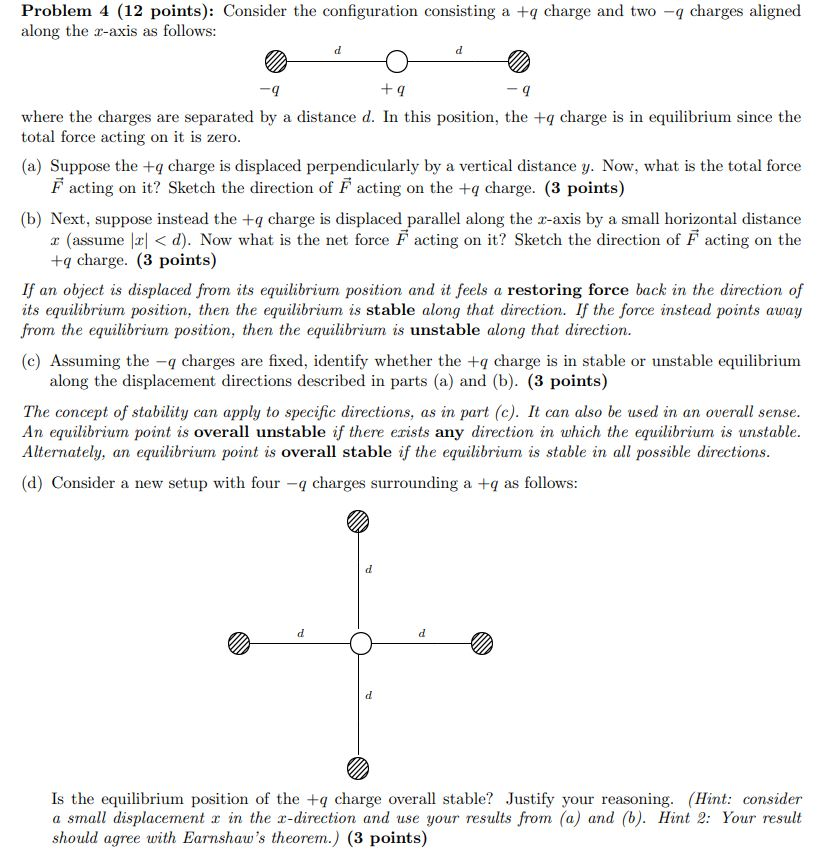# Problem 4 (12 points): Consider the configuration consisting a + charge and two - charges aligned...

###### Question:Problem 4 (12 points): Consider the configuration consisting a + charge and two - charges aligned along the c-axis as follows: -9 +4 -9 where the charges are separated by a distance d. In this position, the + charge is in equilibrium since the total force acting on it is zero. (a) Suppose the + charge is displaced perpendicularly by a vertical distance y. Now, what is the total force F acting on it? Sketch the direction of Facting on the + charge. (3 points) (b) Next, suppose instead the + charge is displaced parallel along the z-axis by a small horizontal distance I assume 3 <d). Now what is the net force Facting on it? Sketch the direction of F acting on the +q charge. (3 points) If an object is displaced from its equilibrium position and it feels a restoring force back in the direction of its equilibrium position, then the equilibrium is stable along that direction. If the force instead points away from the equilibrium position, then the equilibrium is unstable along that direction. (c) Assuming the -q charges are fixed, identify whether the + charge is in stable or unstable equilibrium along the displacement directions described in parts (a) and (b). (3 points) The concept of stability can apply to specific directions, as in part (c). It can also be used in an overall sense. An equilibrium point is overall unstable if there erists any direction in which the equilibrium is unstable. Alternately, an equilibrium point is overall stable if the equilibrium is stable in all possible directions. (d) Consider a new setup with four - charges surrounding a +q as follows: a Is the equilibrium position of the + charge overall stable? Justify your reasoning. (Hint: consider a small displacement r in the e-direction and use your results from (a) and (b). Hint 2: Your result should agree with Earnshaw's theorem.) (3 points)